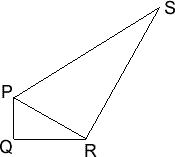You may also likeFitting In

The largest square which fits into a circle is ABCD and EFGH is a square with G and H on the line CD and E and F on the circumference of the circle. Show that AB = 5EF. Similarly the largest equilateral triangle which fits into a circle is LMN and PQR is an equilateral triangle with P and Q on the line LM and R on the circumference of the circle. Show that LM = 3PQLook Before You Leap

Can you spot a cunning way to work out the missing length?Triangle Midpoints

You are only given the three midpoints of the sides of a triangle. How can you construct the original triangle?

Two Right Angles

Age 14 to 16 ShortChallenge LevelIn the figure above, $PQ=2\frac{1}{3}$, $PS = 6\frac{6}{7}$, $PQR$ and $PRS$ are right-angled triangles, and the angles $QPR$ and $RPS$ are the same.

How long is $PR$?

If you liked this problem, here is an NRICH task which challenges you to use similar mathematical ideas.

This problem is taken from the UKMT Mathematical Challenges.
You can find more short problems, arranged by curriculum topic, in our short problems collection.